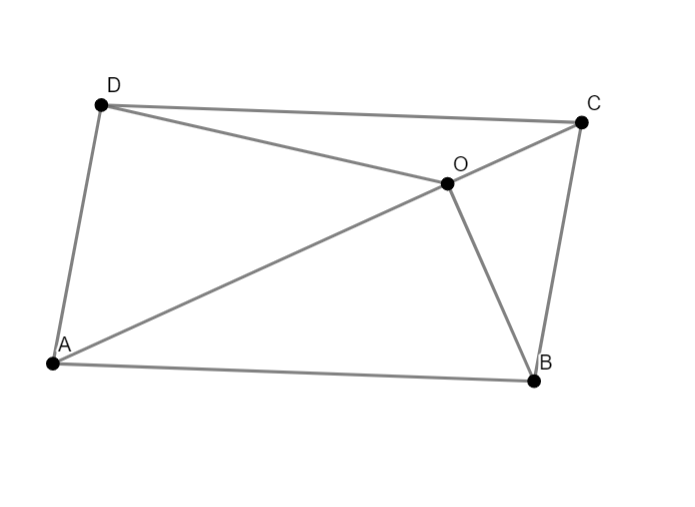Filters
Latest Questions
Mathematics
Diagonals of a parallelogram
If ABCD be a parallelogram and M be the point of intersection of the diagonals. If O is any point then $OA+OB+OC+OD$ is?
1) $3OM$
2) $4OM$
3) $OM$
4) $2OM$
5) $\dfrac{1}{2}OM$

Mathematics
Diagonals of a parallelogram
The equations to a pair of opposite sides of a parallelogram are ${x^2} - 5x + 6 = 0$ and ${y^2} - 6y + 5 = 0$ . The equation to its diagonals are
$A)x + 4y = 13,y = 4x - 7$
$B)4x + y = 13,4y = x - 7$
$C)4x + y = 13,y = 4x - 7$
$D)y - 4x = 13,y + 4x = 7$
Mathematics
Diagonals of a parallelogram
The length of the longest pole that can be put in a room of dimensions 10m×10m×5m is:
(a)15m
(b)16m
(c)10m
(d)12m

Mathematics
Diagonals of a parallelogram
State ‘true’ or ‘false’
The diagonals of a parallelogram bisect each other at right angles.
(a) True
(b) False

Mathematics
Diagonals of a parallelogram
In the parallelogram $ABCD$ , $O$ is any point on diagonal $AC$ . Prove that $ar(\vartriangle OBC)=ar(\vartriangle OCD)$ .Mathematics
Diagonals of a parallelogram
What is the length of a diagonal of a rectangle with length 12 and width 5?
Mathematics
Diagonals of a parallelogram
How do you find the point of intersection of diagonals?

Mathematics
Diagonals of a parallelogram
Three consecutive vertices of a parallelogram are $\left( {1, - 2} \right)$, $\left( {3,6} \right)$ and $\left( {5,10} \right)$. The coordinates of the fourth vertex are
A.$\left( { - 3,2} \right)$
B.$\left( {2, - 3} \right)$
C.$\left( {3,2} \right)$
D.$\left( { - 2, - 3} \right)$
Mathematics
Diagonals of a parallelogram
The vertices of a parallelogram are (3, – 2), (4, 0), (6, – 3), and (5, – 5). The diagonal intersect at a point M. The coordinates of the point M are
Mathematics
Diagonals of a parallelogram
If coordinates of two adjacent vertices of a parallelogram are (3, 2) and (1, 0) and diagonals bisect each other at (-2, 5), find coordinates of the other two vertices?

Mathematics
Diagonals of a parallelogram
If $P(1,2),Q(4,6),R(5,7)$ and then are vertices of parallelogram PQRS then
A.a=b , b=4
B.a=3, b=4
C.a=2, b=3
D.a=-3, b=5

Mathematics
Diagonals of a parallelogram
The diagonal AC of a parallelogram ABCD intersects DP at the point Q, where P is any point on side AB. Prove that $CQ \times PQ = QA \times QD$.
Prev
1
2